# Chapter 11 Area Related to Circles NCERT Exemplar Solutions Exercise 11.3 Class 10 Maths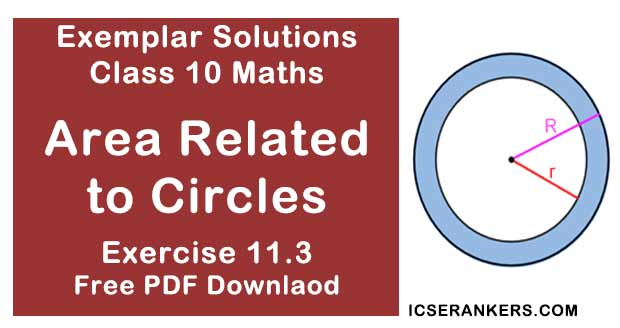Chapter Name NCERT Maths Exemplar Solutions for Chapter 11 Area Related to Circles Exercise 11.3 Book Name NCERT Exemplar for Class 10 Maths Other Exercises Exercise 11.1Exercise 11.2Exercise 11.4 Related Study NCERT Solutions for Class 10 Maths

### Exercise 11.3 Solutions

1. Find the radius of a circle whose circumference is equal to the sum of the circumferences of two circles of radii 15 cm and 18 cm.

Solution

Radius of first circle = r1 = 15 cm
Radius of second circle = r2 = 18 cm
Circumference of 1st circle = 2πr1 = 30π cm
Circumference of 1st circle = 2πr2 = 36π cm
Let the radius of the circle = R
We have,
Circumference of circle = Circumference of first circle + Circumference of second circle
2πR = 2πr1 + 2πr2
⇒ 2πR = 30π + 36π = 66π
⇒ R = 33
Therefore, required radius of a circle is 33 cm.

2. In Fig., a square of diagonal 8 cm is inscribed in a circle. Find the area of the shaded region.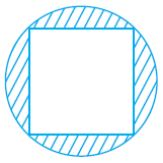Solution
Let us take a be the side of square.
Diameter of a circle = Diagonal of the square = 8 cm
In right angled triangle ABC,
Using Pythagoras theorem,
(AC)2 = (AB)2 + (BC)2
⇒ (8)2 = a2 + a2
⇒ 64 = 2a2
⇒ a2 = 32
Area of square = a2
= 32 cm2
Radius of the circle = Diameter/2
= 4 cm
Area of the circle = πr2
= π(4)2
= 16 cm2
So, the area of the shaded region = Area of circle - Area of square
The area of the shaded region = 16π – 32
= 16 × (22/7) – 32
= 128/7
= 18.286 cm2

3. Find the area of a sector of a circle of radius 28 cm and central angle 45°.

Solution

Area of a sector of a circle = (1/2)r2 θ
(In which r = radius and θ = angle in radians subtended by the arc at the center of the circle)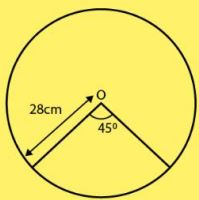We have,
Radius of circle = 28 cm
Angle subtended at the center = 45°
Angle subtended at the center (in radians) = θ
= 45π/180
= π/4
Area of a sector of a circle = (1/2) × r2θ
= (1/2) × (28)2 × (π/4)
= 308 cm2
Therefore, the required area of a sector of a circle is 308 cm2.

4. The wheel of a motor cycle is of radius 35 cm. How many revolutions per minute must the wheel make so as to keep a speed of 66 km/h?

Solution

Radius of wheel = r = 35 cm
1 revolution of the wheel = Circumference of the wheel
= 2πr
= 2 × (22/7) × 35
= 220 cm
Speed of the wheel = 66 km/hr
= (66×1000×100) × 1/60  cm/min
= 110000 cm/min
∴ Number of revolutions in 1 min = 110000/220 = 500
Thus, required number of revolutions per minute is 500.

5. A cow is tied with a rope of length 14 m at the corner of a rectangular field of dimensions 20m × 16m. Find the area of the field in which the cow can graze.

Solution

ABCD is a rectangular field.
Length of field = 20 m
Breadth of the field = 16 m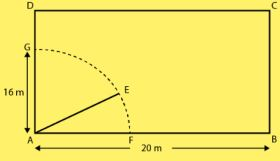A cow is tied at a point A.
Let length of rope be AE = 14 m
Angle subtended at the center of the sector = 90°
Angle subtended at the center (in radians) θ = 90π/180
= π/2
Area of a sector of a circle = (1/2) × r2θ
= (1/2) × (14)2 × π/2
= 154 m2
So, the required area of a sector of a circle is 154 m2.

6. Find the area of the flower bed (with semi-circular ends) shown in Fig.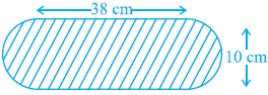Solution

Length and breadth of the rectangular portion AFDC of the flower bed are 38 cm and 10 cm Area of the flower bed = Area of the rectangular portion + Area of the two semi-circles.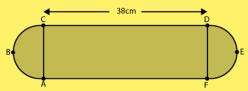Area of rectangle AFDC = Length × Breadth
= 38 × 10
= 380 cm2
Both ends of flower bed are semi-circle in shape.
Diameter of the semi-circle = Breadth of the rectangle AFDC
= 10 cm
Radius of the semi - circle = 10/2  = 5 cm
Area of the semi - circle  = πr2/2
= 25(π/2) cm2
As, there are two semi - circles in the flower bed,
Area of two semi - circles = 2 × ( πr2/2)
= 25π cm2
Therefore, total area of flower bed = (380 + 25π) cm2.

7. In Fig., AB is a diameter of the circle, AC = 6 cm and BC = 8 cm. Find the area of the shaded region (Use π= 3.14).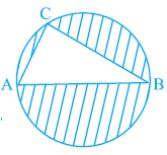Solution
AC = 6 cm and BC = 8 cm
A triangle in a semi - circle with hypotenuse as diameter is right angled triangle.
By Pythagoras theorem in right angled triangle ACB,
(AB)2  = (AC)2  + (CB)2
⇒ AB2  = 62  + 82
⇒ AB2  = 36 + 64
⇒ AB2  = 100
⇒ AB = 10
Diameter of the circle = 10 cm
Radius of the circle = 5 cm
Area of circle = πr2
= π(5)2
= 25π cm2
= 25 × 3.14 cm2
= 78.5 cm2
Area of the right angled triangle = ( 1⁄2 ) × Base × Height
= (1⁄2) × AC × CB
= (1⁄2) × 6 × 8
= 24 cm2
Area of the shaded region = Area of the circle - Area of the triangle
= (78.5 - 24) cm2
= 54.5 cm2

8. Find the area of the shaded field shown in Fig.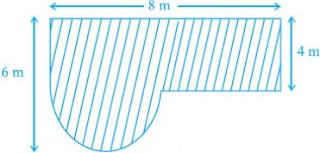Solution

Construction : Join ED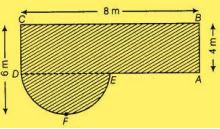Radius of semi - circle DFE = 6 - 2 = 4M
Area of rectangle ABCD  = BC × AB
= 8 × 4 = 32m2
Area of semicircle DFE = πr2/2
= π(2)2/2
= 2π m2
Area of shaded region = Area of rectangle ABCD + Area of semicircle DFE
= (32 + 2π) m2.

9. Find the area of the shaded region in Fig.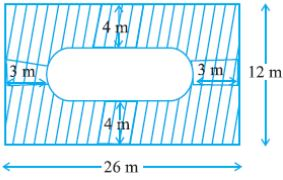Solution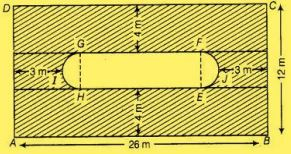Construction: Join GH and EF
Breadth of inner rectangle EFGH =12-(4+4)
= 4 m
Diameter of semicircle EFJ = 4m
Length of inner rectangle EFGH= 26-(5+5)
= 16m
Therefore, area of two semicircles = 2 × πr2/2
= π(2)2/2
= 4π m
Now,
Area of inner rectangle (EFGH) = EH × FG
= 16 × 4
= 64 m2
Area of outer rectangle (ABCD) = 26 × 13
= 312 m2
Area of shaded region = Area of outer rectangle - (area of two semicircles + area of inner rectangles)
= 312 - (64 + 4π)
= 248 - 4π) m2

10. Find the area of the minor segment of a circle of radius 14 cm, when the angle of the corresponding sector is 60°.
Solution
Given,
Radius of circle (r) = 14 cm
Angle of the corresponding sector ,central angle (θ) = 60°
As,
In ΔAOB,
OA = OB = Radius of circle
So,
ΔAOB is isosceles.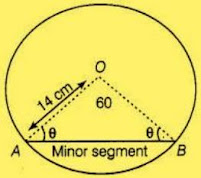Therefore,
Angle (OAB) = Angle (OBA) = θ
In ΔOAB,
AOB + OAB + OBA = 180°
⇒ 60° + θ + θ = 180°
⇒ θ = 60°
Therefore, ΔOABis an equilateral triangle.
Also,
OA = OB = AB = 14cm
Area of ΔOAB = (√3/4) (side)2
= (√3/4) (14)2
= 49√3 cm2

11. Find the area of the shaded region in Fig., where arcs drawn with centres A, B, C and D intersect in pairs at mid-points P, Q, R and S of the sides AB, BC,CD and DA, respectively of a square ABCD (Use π = 3.14).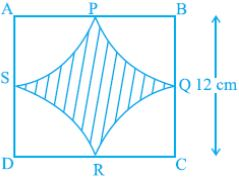Solution
Side of a square BC = 12 cm
Q is a mid - point of BC.
Radius BQ = 12/2 = 6 cm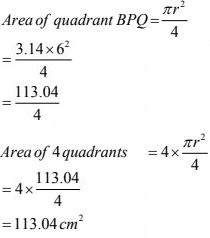Now, Area of square (ABCD) = (12)2
= 144 cm2
Area of shaded region = Area of square - Area of  four quadrants
= 144 - 113.04
= 30.93 cm2

12. In Fig., arcs are drawn by taking vertices A, B and C of an equilateral triangle of side 10 cm. to intersect the sides BC, CA and AB at their respective mid-points D, E and F. Find the area of the shaded region(Use π = 3.14).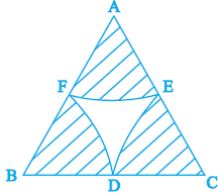Solution
ABC is an equilateral triangle.
And,
AB = BC = CA = 10 cm
As, D, E, F are mid points of the sides,
AE = EC = CD = BD = BF = FA = 5cm
⇒ ∠A = ∠B = ∠C = 60°
Area of sector CDE = θ/360 × πr2
= 60/360 × π(5)2
= 13.0833 cm2
Area of shaded region = 3 × Area of sector CDE
= 3 × 13.0833
= 39.25 cm2

13. In Fig. 11.12, arcs have been drawn with radii 14 cm each and with centres P, Q and R. Find the area of the shaded region.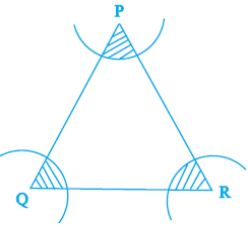Solution
Radii of each arc (r) = 14 cm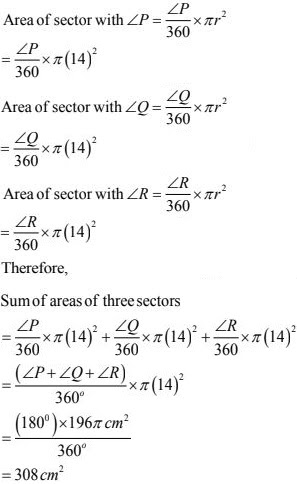14. A circular park is surrounded by a road 21 m wide. If the radius of the park is105 m, find the area of the road.
Solution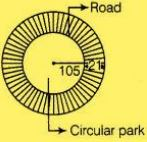A circular park is surrounded by a road,
Radius of park = 105 m
Therefore,
=105+21
=126m
Now,
Area of road = Area of whole circular portion – Area of park
= π(1262 - 1052)
= (22/7) (126 - 105)(126 + 105)
= 15246 m2

15. In Fig., arcs have been drawn of radius 21 cm each with vertices A, B, C and D of quadrilateral ABCD as centres. Find the area of the shaded region.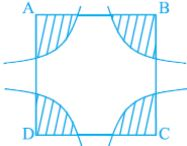Solution
Radius of each arc (r) = 21 cm
Area of sector with ∠A = (∠A/360) × πr2
= (∠A/360) × π(21)2
Area of sector with ∠B = (∠B/360) × πr2
= (∠B/360) × π(21)2
Area of sector with ∠C = (∠C/360) × πr2
= (∠C/360) × π(21)2
Area of sector with ∠D = (∠D/360) × πr2
= (∠D/360) × π(21)2
Therefore,
Sum of areas of four sectors =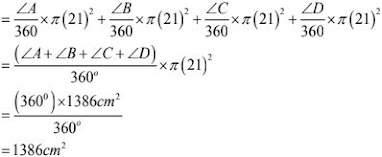16. A piece of wire 20 cm long is bent into the form of an arc of a circle subtending an angle of 60° at its centre. Find the radius of the circle.
Solution
Length of arc of circle  = 20 cm
Central angle (θ) = 60°
Now,
Length of arc = (θ/360) × 2πr
20 = (60/360) × 2πr
⇒ r = 60/π   cm